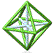# Geometrical Concepts from Constructions, Models, and Investigations Summary

## Tuesday, July 8, 2003

The geometry group continued to explore concepts of spherical geometry, working with inflatable beach balls. People shared how they introduce and develop the concepts of volume and surface area of a sphere, in their classrooms. Direct experimentation and Cavalieri's Principle were discussed as means to derive the formula for volume. Some people start with the volume of a sphere to arrive at the formula for its surface area. For the purposes of our work, we defined the radius of our sphere to be one unit.

Art directed an exploratory lesson examining areas on the sphere. We began by determining the area of a lune of a given angle, measured in radians. We then extended the exploration by bisecting the lune, and tried to arrive at a means of calculating the area of a spherical triangle.

As part of our work, we decided we should discuss the nature of a triangle on the sphere. Part of the discussion, revolved around the fact that on the Euclidean plane, all three vertices of a triangle cannot be co-linear. On the plane, if all three are co-linear, they do not define a closed interior space. On the sphere, three co-linear points, given two are antipodal, may define a closed interior space. This is what we had already been describing as a lune. Now the question became; is it also a triangle? And if so, can a triangle with three 180 degree angles exist on the sphere? Can a spherical triangle have even larger angles?

After developing the relationship between the sum of the angles and the area of a spherical triangle, (You didn't really expect me to give away the answer, did you?!), we looked briefly at parallel transport on the Euclidean plane and on the sphere. "Holonomy" tells how an object is rotated when parallel transported along a closed curve.PCMI@MathForum Home || IAS/PCMI Home© 2001 - 2018 Park City Mathematics Institute IAS/Park City Mathematics Institute is an outreach program of the School of Mathematics at the Institute for Advanced Study, Einstein Drive, Princeton, NJ 08540 Send questions or comments to: Suzanne Alejandre and Jim King

This material is based upon work supported by the National Science Foundation under Grant No. 0314808.
Any opinions, findings, and conclusions or recommendations expressed in this material are those of the author(s) and do not necessarily reflect the views of the National Science Foundation.